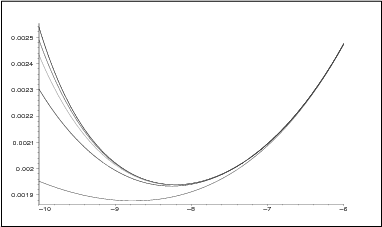]> 5.4.2 Example 2: RC–transmission line

#### 5.4.2 Example 2: $RC$–transmission line

Consider the problem of parametric optimization of the system consisting of a proportional controller (operational ampliﬁer) and a distributed parameter plant (the $RC$–transmission line) discussed in Chapter 2. It relies on ﬁnding minimum of the function

$J\left(K\right)={∥f∥}_{{\text{L}}^{2}\left(0,\infty \right)}^{2}$

in the interval $\left(-cosh\pi ,1\right)$ being the stability region in the space of the parameter $K$ and the function $f$ is given by its Laplace transform

 $\stackrel{̂}{f}\left(s\right)=\frac{E\left(cosh\sqrt{s}-1\right)}{s\left(1-K\right)\left(cosh\sqrt{s}-K\right)}$ (5.12)

In Section 2.7, to solve the problem we employed spectral properties of the operator describing the closed–loop system in the state space ${\text{L}}^{2}\left(0,1\right)$. It was shown that the formula expressing the performance index as a function of $K$ has the form (2.53). Recall that the formula (2.53) admits a simple, highly stable computer implementation which is due to the fast convergence of the series in (2.53).

The efforts to implement a reasonable approximation of ${∥{P}_{n}f∥}_{{\text{L}}^{2}\left(0,\infty \right)}^{2}$ with the aid of both (5.4) and (5.10) failed down on Matlab 6 out of ill–conditioning. Recall that the standard Matlab calculations are carried on $32$ positions. Therefore to control the accuracy of ﬂoating–point calculations the formula (5.10) was implemented on Maple 6 rather than on Matlab 6. This was achieved by Digits:=90; command. The comparison of the exact values of the performance index (2.53) with their approximations are depicted in Figure 5.3.Figure 5.3: Plots of the performance index for the system examined in Example 2. The upper curve is exact, the lower ones are the approximations for $n=20$, $n=30$, $n=40$ and $n=50$